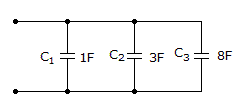# Electronics - Capacitors - Discussion

### Discussion :: Capacitors - General Questions (Q.No.7)

7.

What is the total capacitance?[A]. 1 F [B]. 12 F [C]. 0.615 F [D]. 8 F

Explanation:

No answer description available for this question.

 Narendra said: (Oct 22, 2010) When the capacitors are in parallel then the capacintance =c1+c2+c3 Therefore, capacitance =1 + 3 + 8 = 12 F

 M.V.Krishna said: (Sep 3, 2011) When capacitors are in parallel:. C = c1+c2+c3+...

 Vijaya Veenam said: (Feb 23, 2012) If the capacitors are in series then total capacitance is (1/c)=(1/c1)+(1/c2)+(1/c3)+..... If the capacitors are in parallel then total capacitance is c=c1+c2+c3+...... If the resistors are in series then total resistance is R=R1+R2+R3+..... If the resistors are in PARALLEL then total resistance is (1/R)=(1/R1)+(1/R2)+(1/R3)+.....

 Vpc said: (Jul 7, 2012) If all the positive ends are connected to one end and all the negative ends are connected to one end then the connection is said to be parallel connection and if the positive end of first capacitor is connected to the negative end of second and positive of second to negative of third and so on and the positive end of last connected to negative end of first then the capacitors are said to be in series.

 Kishan Tj said: (Aug 29, 2012) When the capacitors are in parallel then the capacintance =c1+c2+c3 Therefore, capacitance =1 + 3 + 8 = 12 F

 Arzoo said: (Sep 11, 2012) When capacitors are said to be connected in parallel then the total capacitance can be measured by adding them, i.e., c1+c2+c3=1+3+8=12F.

 Ankur Pandey said: (Apr 10, 2013) If all the +ve ends are connected to one and all the -ve ends are connected to one end, the connection is said to be Parallel connection. So This question can solve by this way C=C1+C2+C3. Thus, 1+3+8=12F.

 Gopal Shukla said: (Jul 16, 2016) c1 + c2 + c3 = 1 + 3 + 8 = 12.

 Rakshana said: (Aug 1, 2017) I agree @Kishan Tj.